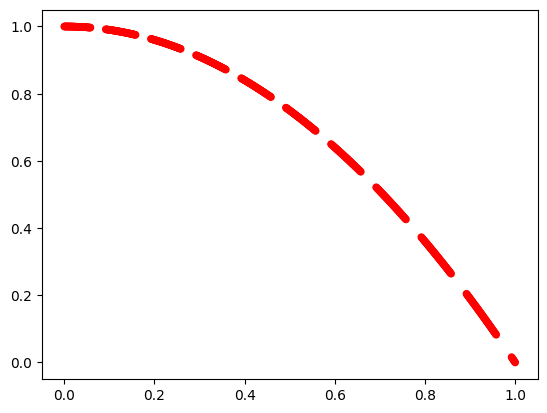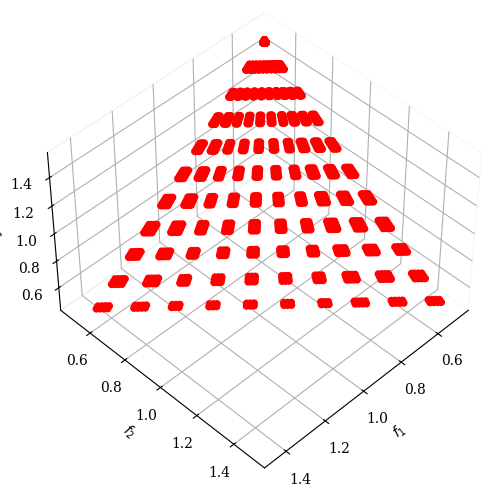Version: 0.6.0

# DAS-CMOP¶

DAS-CMOP is a constrained multi-objective test suite with tunable constraints . The constraints are tuned using a difficulty triplet: $$(\eta, \zeta, \gamma)$$, with $$\eta, \zeta, \gamma \in [0, 1]$$. The triplet allows to adjust the diversity, the feasibility and the convergence hardness respectively.

There are 6 biobjective problems DAS-CMOP1-6 (11 constraints) and 3 three-objective problems DAS-CMOP7-9 (7 constraints). Each of these can be initialized with a custom difficulty triplet or the authors proposed a set of 16 triplets:

No.

Difficulty

No.

Difficulty

No

Difficulty

No

Difficulty

1

(0.25,0.0,0.0)

2

(0.0,0.25,0.0)

3

(0.0,0.0,0.25)

4

(0.25,0.25,0.25)

5

(0.5,0.0,0.0)

6

(0.0,0.5,0.0)

7

(0.0,0.0,0.5)

8

(0.5,0.5,0.5)

9

(0.75,0.0,0.0)

10

(0.0,0.75,0.0)

11

(0.0,0.0,0.75)

12

(0.75,0.75,0.75)

13

(0.0,1.0,0.0)

14

(0.5,1.0,0.0)

15

(0.0,1.0,0.5)

16

(0.5,1.0,0.5)

The Pareto fronts are different for each triplet.

## DAS-CMOP1 (1)¶

:

from pymoo.problems import get_problem
from pymoo.util.plotting import plot

problem = get_problem("dascmop1", 1)
plot(problem.pareto_front(), no_fill=True)## DAS-CMOP7 (12)¶

:

from pymoo.problems import get_problem
from pymoo.visualization.scatter import Scatter

pf = get_problem("dascmop7", 12).pareto_front()

:

<pymoo.visualization.scatter.Scatter at 0x7fd3ac712e80>:

from pymoo.problems import get_problem
from pymoo.visualization.scatter import Scatter

pf = get_problem("dascmop7", 12).pareto_front()

:

<pymoo.visualization.scatter.Scatter at 0x7fd35eabdcd0>